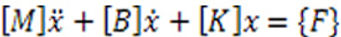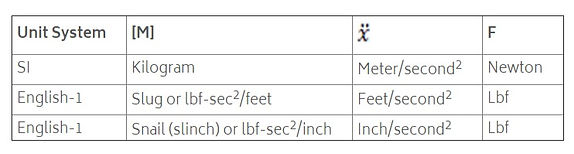## UNITS IN NASTRAN ARE USER DEFINED

Since no unit system is defined in a NASTRAN run it is the responsibility of the user to use consistent units. In general this means each matrix product below should produce a force unit:## SOME COMMONLY USED CONSISTENT UNIT SYSTEMS ARE:## FOR EXAMPLE, CONSISTENT UNITS ON JUST THE TERM TO PRODUCE A FORCE UNIT WOULD BE:## GLOBAL CHANGES TO MASS UNITS

NASTRAN does allow a global change in Mass units with the parameter WTMASS. This is typically used when using English units so the user can enter concentrated masses, densities, etc with weight units since this is easier to think in terms of weight than actual English mass units. NASTRAN will then multiple the assembled structural mass matrix by the value on the PARAM WTMASS card hence converting the weight to mass. The relationship of mass to weight is: weight= mass*gravity. So to convert weight to mass is 1/gravity. Applicable WTMASS values would be: